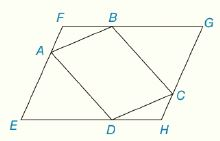Chapter 4.CR, Problem 28CRElementary Geometry For College St...

7th Edition
Alexander + 2 others
ISBN: 9781337614085

Solutions

Chapter
SectionElementary Geometry For College St...

7th Edition
Alexander + 2 others
ISBN: 9781337614085
Textbook Problem

Review Exercises Given: ∆ F A B ≅ ∆ H C D ∆ E A D ≅ ∆ G C B Prove: A B C D is a ▱To determine

To Prove:

The provided quadrilateral ABCD is a parallelogram.

Explanation

Proof:

Theorem on parallelogram:

If both pairs of opposite sides of a quadrilateral are congruent, then the quadrilateral is a parallelogram.

Description:

It is given that EFGH is a quadrilateral with FABHCD and EADGCB.

Since FABHCD, its sides must be equal in measures. That is, FA=HC, AB=CD and BF=DH.

Since EADGCB, its sides must be equal in measures

Still sussing out bartleby?

Check out a sample textbook solution.

See a sample solution

The Solution to Your Study Problems

Bartleby provides explanations to thousands of textbook problems written by our experts, many with advanced degrees!

Get Started

Prove the statement using the , definition of a limit. limx2(x2+2x7)=1

Single Variable Calculus: Early Transcendentals, Volume I

Evaluate the iterated integral: 1122(x2y2)dydx

Calculus: Early Transcendental Functions

In problems 17-30, simplify each expression so that only positive exponents remain. 22.

Mathematical Applications for the Management, Life, and Social Sciences

Fill in each blank: 3tbs=tsp

Elementary Technical Mathematics

Evaluate the integral. 27. x2+2xdx

Single Variable Calculus: Early Transcendentals

By definition the improper integral It is not an improper integral.

Study Guide for Stewart's Single Variable Calculus: Early Transcendentals, 8th

The third partial sum of is:

Study Guide for Stewart's Multivariable Calculus, 8th